# The ARIMA Procedure

### The ESACF Method

The extended sample autocorrelation function (ESACF) method can tentatively identify the orders of a stationary or nonstationary ARMA process based on iterated least squares estimates of the autoregressive parameters. Tsay and Tiao (1984) proposed the technique, and Choi (1992) provides useful descriptions of the algorithm.

Given a stationary or nonstationary time series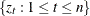with mean corrected form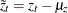with a true autoregressive order of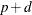and with a true moving-average order of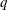, you can use the ESACF method to estimate the unknown ordersandby analyzing the autocorrelation functions associated with filtered series of the form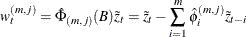where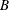represents the backshift operator, where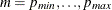are the autoregressive test orders, where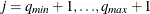are the moving-average test orders, and where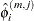are the autoregressive parameter estimates under the assumption that the series is an ARMA(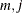) process.

For purely autoregressive models (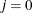), ordinary least squares (OLS) is used to consistently estimate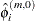. For ARMA models, consistent estimates are obtained by the iterated least squares recursion formula, which is initiated by the pure autoregressive estimates: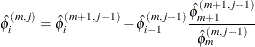Theth lag of the sample autocorrelation function of the filtered series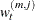is the extended sample autocorrelation function, and it is denoted as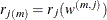.

The standard errors of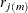are computed in the usual way by using Bartlett’s approximation of the variance of the sample autocorrelation function,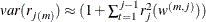.

If the true model is an ARMA (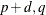) process, the filtered seriesfollows an MA() model for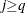so that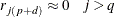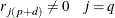Additionally, Tsay and Tiao (1984) show that the extended sample autocorrelation satisfies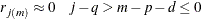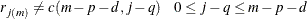where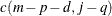is a nonzero constant or a continuous random variable bounded by –1 and 1.

An ESACF table is then constructed by using thefor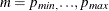andto identify the ARMA orders (see Table 7.4). The orders are tentatively identified by finding a right (maximal) triangular pattern with vertices located at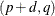and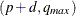and in which all elements are insignificant (based on asymptotic normality of the autocorrelation function). The vertexidentifies the order. Table 7.5 depicts the theoretical pattern associated with an ARMA(1,2) series.

Table 7.4: ESACF Table

 MA AR 0 1 2 30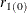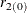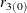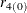1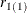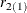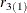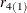2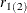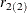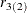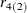3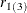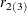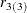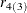Table 7.5: Theoretical ESACF Table for an ARMA(1,2) Series

 MA AR 0 1 2 3 4 5 6 7 0 * X X X X X X X 1 * X 0 0 0 0 0 0 2 * X X 0 0 0 0 0 3 * X X X 0 0 0 0 4 * X X X X 0 0 0 X = significant terms 0 = insignificant terms * = no pattern